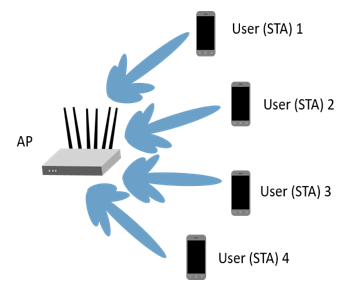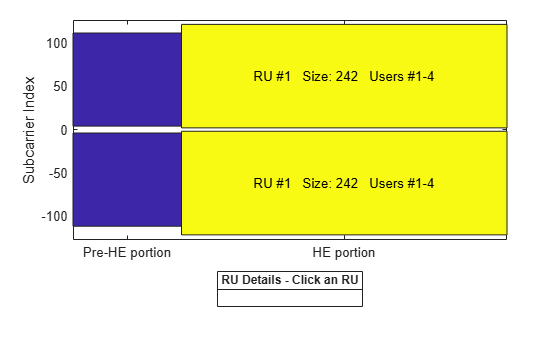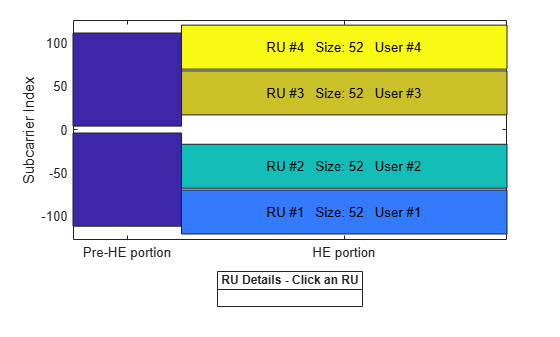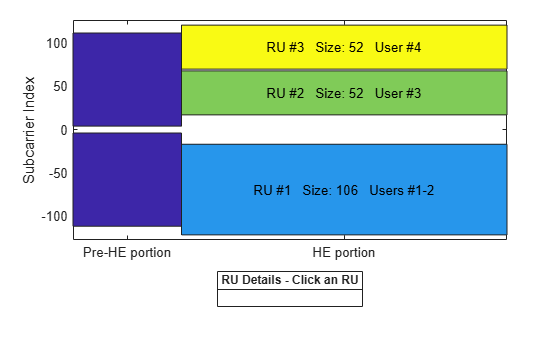# 802.11ax Downlink OFDMA and Multi-User MIMO Throughput Simulation

This example shows the transmit and receive processing for an IEEE® 802.11ax™ multi-user downlink transmission over a TGax indoor fading channel. Three transmission modes are simulated: OFDMA, MU-MIMO, and a combination of OFDMA and MU-MIMO.

### Introduction

This example simulates a scenario of an access point (AP) transmitting to four stations (STAs) simultaneously using high efficiency (HE) multi-user (MU) format packets as specified in IEEE P802.11ax™/D4.1 [ 1 ].The HE multi-user format can be configured for an OFDMA transmission, a MU-MIMO transmission, or a combination of the two. This flexibility allows an HE-MU packet to transmit to a single user over the whole band, multiple users over different parts of the band (OFDMA), or multiple users over the same part of the band (MU-MIMO).

Three transmission modes are compared for the multi-user downlink scenario:

1. OFDMA - each of the four users is assigned a separate resource unit (RU) and the transmission is beamformed.

2. MU-MIMO - all four users share the full band.

3. Mixed MU-MIMO and OFDMA - Two users share a single RU in a MU-MIMO configuration, and the remaining two users are assigned a single RU each.

For a detailed overview of 802.11ax formats, see the 802.11ax Parameterization for Waveform Generation and Simulation example.

For each transmission mode, the AP transmits a burst of 10 packets, and each STA demodulates and decodes the data intended for it. An evolving TGax indoor MIMO channel with AWGN is modeled between the AP and each STA. The raw AP throughput is provided as a metric to compare the transmission modes and is calculated by counting the number of packets transmitted successfully to all STAs. The simulation is repeated for different path losses. In this example, all the transmissions are beamformed. Therefore, before simulating the data transmission, the channel between the AP and each station is sounded under perfect conditions to obtain channel state information.

### Transmission Configuration

An `wlanHEMUConfig` object is used to configure the transmission of an HE-MU packet. Three transmission configuration objects are specified to define the three different AP transmissions:

1. `cfgMUMIMO` is a MU-MIMO configuration which consists of a single 242-tone RU with 4 users. Each user has one space-time stream.

2. `cfgOFDMA` is an OFDMA configuration which consists of four 52-tone RUs, each with one user. Each user has two space-time streams.

3. `cfgMixed` is a mixed OFDMA and MU-MIMO configuration which consists of one 106-tone RU shared by two users, and two 52-tone RUs, each with one user. The MU-MIMO users each have one space-time stream, and the OFDMA users each have two space-time streams.

A 20 MHz channel bandwidth is used for all transmissions. Other transmission parameters such as the APEPLength and MCS are the same for all users in all configurations.

First, the MU-MIMO configuration is defined. The allocation index `195` defines a single 242-tone RU, with four users in MU-MIMO. For a description of selecting an allocation index, see the 802.11ax Parameterization for Waveform Generation and Simulation example.

```% MU-MIMO configuration - 4 users on one 242-tone RU cfgMUMIMO = wlanHEMUConfig(195); ```

The allocation plot shows a single RU is assigned to all four users.

```showAllocation(cfgMUMIMO); ```The transmission parameters for each user are now configured.

```numTx = 6; % Number of transmit antennas guardInterval = 0.8; % Guard interval in Microseconds % Configure common parameters for all users cfgMUMIMO.NumTransmitAntennas = numTx; cfgMUMIMO.GuardInterval = guardInterval; % Configure per user parameters % STA #1 cfgMUMIMO.User{1}.NumSpaceTimeStreams = 1; cfgMUMIMO.User{1}.MCS = 4; cfgMUMIMO.User{1}.APEPLength = 1000; % STA #2 cfgMUMIMO.User{2}.NumSpaceTimeStreams = 1; cfgMUMIMO.User{2}.MCS = 4; cfgMUMIMO.User{2}.APEPLength = 1000; % STA #3 cfgMUMIMO.User{3}.NumSpaceTimeStreams = 1; cfgMUMIMO.User{3}.MCS = 4; cfgMUMIMO.User{3}.APEPLength = 1000; % STA #4 cfgMUMIMO.User{4}.NumSpaceTimeStreams = 1; cfgMUMIMO.User{4}.MCS = 4; cfgMUMIMO.User{4}.APEPLength = 1000; ```

Next the OFDMA configuration is defined. The allocation index `112` defines four 52-tone RUs, each serving a single user.

```% OFDMA configuration - 4 users, each on a 52-tone RU cfgOFDMA = wlanHEMUConfig(112); ```

The allocation plot shows the four RUs, each with a single user. When comparing this allocation plot to the full band MU-MIMO plot, it is apparent that the total number of subcarriers used (4x52 = 208 subcarriers) is less than the MU-MIMO allocation (242 subcarriers). The fewer number of subcarriers allow guards between each OFDMA user.

```showAllocation(cfgOFDMA); ```The transmission parameters for each user are now configured.

```% Configure common parameters for all users cfgOFDMA.NumTransmitAntennas = numTx; cfgOFDMA.GuardInterval = guardInterval; % Configure per user parameters % STA #1 (RU #1) cfgOFDMA.User{1}.NumSpaceTimeStreams = 2; cfgOFDMA.User{1}.MCS = 4; cfgOFDMA.User{1}.APEPLength = 1000; % STA #2 (RU #2) cfgOFDMA.User{2}.NumSpaceTimeStreams = 2; cfgOFDMA.User{2}.MCS = 4; cfgOFDMA.User{2}.APEPLength = 1000; % STA #3 (RU #3) cfgOFDMA.User{3}.NumSpaceTimeStreams = 2; cfgOFDMA.User{3}.MCS = 4; cfgOFDMA.User{3}.APEPLength = 1000; % STA #4 (RU #4) cfgOFDMA.User{4}.NumSpaceTimeStreams = 2; cfgOFDMA.User{4}.MCS = 4; cfgOFDMA.User{4}.APEPLength = 1000; ```

Finally, the mixed MU-MIMO and OFDMA configuration is defined. The allocation index `25` defines a 106-tone RU with two users, and two 52-tone RUs, each with one user.

```% Mixed OFDMA and MU-MIMO configuration cfgMixed = wlanHEMUConfig(25); ```

The allocation plot shows the three RUs, one with 2 users (MU-MIMO), and the others with one user each (OFDMA).

```showAllocation(cfgMixed); ```The transmission parameters for each user are now configured.

```% Configure common parameters for all users cfgMixed.NumTransmitAntennas = numTx; cfgMixed.GuardInterval = guardInterval; % Configure per user parameters % RU #1 has two users (MU-MIMO) and a total of 2 STS (1 per user) % STA #1 (RU #1) cfgMixed.User{1}.NumSpaceTimeStreams = 1; cfgMixed.User{1}.MCS = 4; cfgMixed.User{1}.APEPLength = 1000; % STA #2 (RU #1) cfgMixed.User{2}.NumSpaceTimeStreams = 1; cfgMixed.User{2}.MCS = 4; cfgMixed.User{2}.APEPLength = 1000; % The remaining two users are OFDMA % STA #3 (RU #2) cfgMixed.User{3}.NumSpaceTimeStreams = 2; cfgMixed.User{3}.MCS = 4; cfgMixed.User{3}.APEPLength = 1000; % STA #4 (RU #3) cfgMixed.User{4}.NumSpaceTimeStreams = 2; cfgMixed.User{4}.MCS = 4; cfgMixed.User{4}.APEPLength = 1000; ```

### Channel Model Configuration

A TGax indoor channel model is used in this example. An individual channel is used to simulate the link between the AP and each user. A TGax channel object, `tgaxBase` is created with properties relevant for all users. In this example, the delay profile (Model-D) and number of receive antennas are common for all users. Model-D is considered non-line of sight when the distance between transmitter and receiver is greater than or equal to 10 meters. This is described further in `wlanTGaxChannel`. A fixed seed is used for the channel to allow repeatability.

```% Create channel configuration common for all users tgaxBase = wlanTGaxChannel; tgaxBase.DelayProfile = 'Model-D'; % Delay profile tgaxBase.NumTransmitAntennas = numTx; % Number of transmit antennas tgaxBase.NumReceiveAntennas = 2; % Each user has two receive antennas tgaxBase.TransmitReceiveDistance = 10; % Non-line of sight distance tgaxBase.ChannelBandwidth = cfgMUMIMO.ChannelBandwidth; tgaxBase.SampleRate = wlanSampleRate(cfgMUMIMO); % Set a fixed seed for the channel tgaxBase.RandomStream = 'mt19937ar with seed'; tgaxBase.Seed = 5; ```

Next a channel is created for each user. The channel for each user is a clone of the `tgaxBase`, but with a unique `UserIndex` property, and is stored in a cell array `tgax`. The `UserIndex` property of each individual channel is set to provide a unique channel for each user. The resultant channels are used in the simulation as shown below.```% A cell array stores the channel objects, one per user numUsers = numel(cfgMixed.User); % Number of users simulated in this example tgax = cell(1,numUsers); % Generate per-user channels for userIdx = 1:numUsers tgax{userIdx} = clone(tgaxBase); tgax{userIdx}.UserIndex = userIdx; % Set unique user index end ```

### Beamforming Feedback

Transmit beamforming for both OFDMA and MU-MIMO relies on knowledge of the channel state between transmitter and receiver at the beamformer. Feedback of the per-subcarrier channel state is provided by each STA by channel sounding. A null data packet (NDP) is transmitted by the AP, and each STA uses this packet to determine the channel state. The channel state is then fed-back to the AP. The same process is used for 802.11ac™ in the 802.11ac Transmit Beamforming and 802.11ac Multi-User MIMO Precoding examples, but an HE single user NDP packet is used instead of a VHT packet. In this example, the feedback is considered perfect; there is no noise present for channel sounding and the feedback is uncompressed. The `heUserBeamformingFeedback` helper function detects the NDP and uses channel estimation to determine the channel state information. Singular value decomposition (SVD) is then used to calculate the beamforming feedback.

```% Create an NDP with the correct number of space-time streams to generate % enough LTF symbols cfgNDP = wlanHESUConfig('APEPLength',0,'GuardInterval',0.8); % No data in an NDP cfgNDP.ChannelBandwidth = tgaxBase.ChannelBandwidth; cfgNDP.NumTransmitAntennas = cfgMUMIMO.NumTransmitAntennas; cfgNDP.NumSpaceTimeStreams = cfgMUMIMO.NumTransmitAntennas; % Generate NDP packet - with an empty PSDU as no data txNDP = wlanWaveformGenerator([],cfgNDP); % For each user STA, pass the NDP packet through the channel and calculate % the feedback channel state matrix by SVD. staFeedback = cell(1,numUsers); for userIdx = 1:numel(tgax) % Received waveform at user STA with 50 sample padding. No noise. rx = tgax{userIdx}([txNDP; zeros(50,size(txNDP,2))]); % Get the full-band beamforming feedback for a user staFeedback{userIdx} = heUserBeamformingFeedback(rx,cfgNDP); end ```

### Simulation Parameters

Different path losses are simulated in this example. The same path loss and noise floor is applied to all users. For each path loss simulated, 10 packets are passed through the channel. Packets are separated by 20 microseconds.

```cfgSim = struct; cfgSim.NumPackets = 10; % Number of packets to simulate for each path loss cfgSim.Pathloss = (96:3:105); % Path losses to simulate in dB cfgSim.TransmitPower = 30; % AP transmit power in dBm cfgSim.NoiseFloor = -89.9; % STA noise floor in dBm cfgSim.IdleTime = 20; % Idle time between packets in us ```

### Simulation with OFDMA

The scenario is first simulated with the OFDMA configuration and transmit beamforming.

The steering matrix for each RU is calculated using the feedback from the STAs. The `heMUCalculateSteeringMatrix` helper function calculates the beamforming matrix for an RU given the CSI feedback.

```% For each RU, calculate the steering matrix to apply for ruIdx = 1:numel(cfgOFDMA.RU) % Calculate the steering matrix to apply to the RU given the feedback steeringMatrix = heMUCalculateSteeringMatrix(staFeedback,cfgOFDMA,cfgNDP,ruIdx); % Apply the steering matrix to each RU cfgOFDMA.RU{ruIdx}.SpatialMapping = 'Custom'; cfgOFDMA.RU{ruIdx}.SpatialMappingMatrix = steeringMatrix; end ```

The `heMUSimulateScenario` helper function performs the simulation. The pre-HE preamble of 802.11ax is backwards compatible with 802.11ac, therefore in this example the front-end synchronization components for a VHT waveform are used to synchronize the HE waveform at each STA. For each packet and path loss simulated the following processing steps occur:

1. A PSDU is created and encoded to create a single packet waveform.

2. The waveform is passed through an evolving TGax channel model and AWGN is added to the received waveform. The channel state is maintained between packets.

3. The packet is detected.

4. Coarse carrier frequency offset is estimated and corrected.

5. Fine timing synchronization is established.

6. Fine carrier frequency offset is estimated and corrected.

7. The HE-LTF is extracted from the synchronized received waveform. The HE-LTF is OFDM demodulated and channel estimation is performed.

8. The HE Data field is extracted from the synchronized received waveform and OFDM demodulated.

9. Common pilot phase tracking is performed to track any residual carrier frequency offset.

10. The phase corrected OFDM symbols are equalized with the channel estimate.

11. Noise estimation is performed using the demodulated data field pilots and single-stream channel estimate at pilot subcarriers.

12. The equalized symbols are demodulated and decoded to recover the PSDU.

13. The recovered PSDU is compared to the transmitted PSDU to determine if the packet has been recovered successfully.

The simulation is run for the OFDMA configuration.

```disp('Simulating OFDMA...'); throughputOFDMA = heMUSimulateScenario(cfgOFDMA,tgax,cfgSim); ```
```Simulating OFDMA... Pathloss 96.0 dB, AP throughput 66.1 Mbps Pathloss 99.0 dB, AP throughput 66.1 Mbps Pathloss 102.0 dB, AP throughput 49.6 Mbps Pathloss 105.0 dB, AP throughput 16.5 Mbps ```

### Simulation with MU-MIMO

Now the scenario is simulated with the MU-MIMO configuration. The `heMUCalculateSteeringMatrix` helper function calculates the beamforming matrix for an RU given the CSI feedback for all users in the MU-MIMO allocation. A zero forcing solution is used to calculate the steering matrix within the helper function.

```% Calculate the steering matrix to apply to the RU given the feedback ruIdx = 1; % Index of the one and only RU steeringMatrix = heMUCalculateSteeringMatrix(staFeedback,cfgMUMIMO,cfgNDP,ruIdx); % Apply the steering matrix to the RU cfgMUMIMO.RU{1}.SpatialMapping = 'Custom'; cfgMUMIMO.RU{1}.SpatialMappingMatrix = steeringMatrix; ```

Run the simulation for the MU-MIMO configuration.

```disp('Simulating MU-MIMO...'); throughputMUMIMO = heMUSimulateScenario(cfgMUMIMO,tgax,cfgSim); ```
```Simulating MU-MIMO... Pathloss 96.0 dB, AP throughput 110.5 Mbps Pathloss 99.0 dB, AP throughput 110.5 Mbps Pathloss 102.0 dB, AP throughput 71.8 Mbps Pathloss 105.0 dB, AP throughput 0.0 Mbps ```

### Simulation with Combined MU-MIMO and OFDMA

Finally, the scenario is simulated with the combined MU-MIMO and OFDMA configuration.

The steering matrix for each RU is calculated using the feedback from the STAs, including the MU-MIMO RU. The `heMUCalculateSteeringMatrix` helper function calculates the beamforming matrix for an RU given the CSI feedback.

```% For each RU calculate the steering matrix to apply for ruIdx = 1:numel(cfgMixed.RU) % Calculate the steering matrix to apply to the RU given the feedback steeringMatrix = heMUCalculateSteeringMatrix(staFeedback,cfgMixed,cfgNDP,ruIdx); % Apply the steering matrix to each RU cfgMixed.RU{ruIdx}.SpatialMapping = 'Custom'; cfgMixed.RU{ruIdx}.SpatialMappingMatrix = steeringMatrix; end ```

Run the simulation for the combined MU-MIMO and OFDMA configuration.

```disp('Simulating Mixed MU-MIMO and OFDMA...'); throughputMixed = heMUSimulateScenario(cfgMixed,tgax,cfgSim); ```
```Simulating Mixed MU-MIMO and OFDMA... Pathloss 96.0 dB, AP throughput 66.1 Mbps Pathloss 99.0 dB, AP throughput 66.1 Mbps Pathloss 102.0 dB, AP throughput 66.1 Mbps Pathloss 105.0 dB, AP throughput 47.9 Mbps ```

### Plot Results

The raw AP throughput for each transmission mode is plotted. The results show for this channel realization at high SNRs (low pathloss) the throughput provided by the MU-MIMO configuration exceeds OFDMA configuration. The packet duration of the MU-MIMO configuration is roughly half that of the OFDMA configuration which provides the throughput gain. As the SNR decreases, the noise dominates and transmit beamforming with OFDMA becomes more effective. The performance of the combined MU-MIMO and OFDMA configuration follow a similar trend to the OFDMA configuration as the packet duration is the same. The performance differs due to different RU sizes and number of space-time streams.

```% Sum throughput for all STAs and plot for all configurations figure; plot(cfgSim.Pathloss,sum(throughputOFDMA,2),'-x'); hold on; plot(cfgSim.Pathloss,sum(throughputMUMIMO,2),'-o'); plot(cfgSim.Pathloss,sum(throughputMixed,2),'-s'); grid on; xlabel('Pathloss (dB)'); ylabel('Throughput (Mbps)'); legend('OFDMA','MU-MIMO','MU-MIMO & OFDMA'); title('Raw AP Throughput'); ```### Appendix

This example uses these helper functions.

### Selected Bibliography

1. IEEE P802.11ax™/D4.1 Draft Standard for Information technology - Telecommunications and information exchange between systems - Local and metropolitan area networks - Specific requirements - Part 11: Wireless LAN Medium Access Control (MAC) and Physical Layer (PHY) Specifications - Amendment 6: Enhancements for High Efficiency WLAN.

## Support평가판 신청